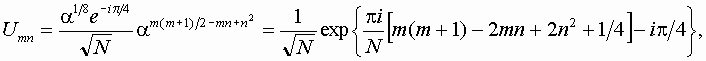From Heisenberg to Schrodinger

In the Heisenberg representation, the shift operators K and A evolve in time: at the next step we have K'=KA, A'=AKA. Let |y> be some state vector. Let us try to construct such an unitary operator U to have in the space of transformed vectors |y'>=U|y> the operators K' and A' equal to original ones, i.e. UK'U-1=K, UA'U-1=A. This is a passage to the Schrodinger representation: a state vector is regarded as evolving in time in accordance with the equation |y'>=U|y>, while the operators K and A are fixed. From the above expression it follows that UK'=KU, UA'=AU, or, in the matrix form, accounting the postulated form of the shift operators

Um+1,n+1=Umnan+1, Um,n+1=Umna2n-m+1.

Departing from the matrix element U00, we obtain step by step the diagonal elements from the first equation: Um+1,m+1=Ummam+1, i.e. Um,m=U00am(m+1)/2. Next, knowing one element in each row, with the second equation obtain the elements of the whole row: Um,n=U00am(m+1)/2-n(m-n).

To make the operator U unitary, we select U00 in such way that |detU|=1. It may be shown that this is ensured if |U00|=1/N1/2. The phase factor may be arbitrary as it does not effect on the observable values (it determines only the origin for quasienergy). Nevertheless, it is convenient to concretize it to have the trace of the matrix equal to real positive number. Thus,причем detU=1 и TrU=1. В частности, для N=5• Classic Arnold's cat map and other torus maps.
• What does it mean periodicity in respect to position and momentum in quantum mechanics?
• Quantum cat map in terms of shift operators. Quantum period.
• From Heisenberg to Schrodinger.
• Dynamics of state vector - computer illustrations and analytic consideration.
• Quantum dynamics in phase space: Husimi distribution and Wigner function.
• Spectrum of quasienergies
• Saratov group
of theoretical nonlinear
dynamics
Хостинг от uCoz